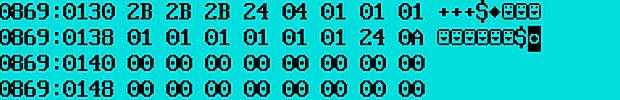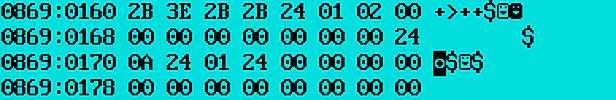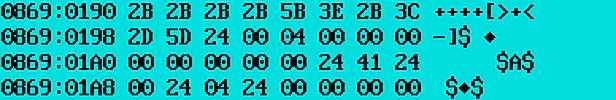Хабрахабр

# Low-level Brainfuck

Building a Brainfuck translator in TurboAssembler.

To begin with, we will write an interpreter in a high-level language, for example, in Pascal.

Let's write a program that outputs a character whose ascii-code corresponds to the number of +

Therefore, we only need the bf-commands + and .

``` var data_mem: array[1..10] of integer; // data array command_mem: string; // command array i: integer; // index of command array j: integer; // index of data array begin j:=1; readln(command_mem); for i:=1 to length(command_mem) do begin // in the cycle we process the string if (command_mem[i]='+') then data_mem[j]:= data_mem[j]+1; if (command_mem[i]='.') then write(chr(data_mem[j])); end; end. ```

bf-code +++++++++++++++++++++++++++++++++. will issue !
(the ascii-code of the symbol ! is 33).

The efficiency of the program can be checked in online ide ideone.com.
Next, replace for operator with goto operator and add the bf-commands — < > .

At the end, we will output the data array data_mem

``` LABEL prev,next; var data_mem: array[1..10] of integer; command_mem: string; i,j,k: integer; begin j:=1; i:=1; readln(command_mem); prev: if i>length(command_mem) then goto next; if (command_mem[i]='+') then data_mem[j]:= data_mem[j]+1; if (command_mem[i]='-') then data_mem[j]:= data_mem[j]-1; if (command_mem[i]='>') then j:=j+1; if (command_mem[i]='<') then j:=j-1; if (command_mem[i]='.') then write(chr(data_mem[j])); i:=i+1; goto prev; next: for k:=1 to 10 do begin write(data_mem[k]); write(' '); end; end. ```

ideone.com

Next, let's add [ and ]

Add another variable i_stor for bracket [ ] loop.

If the current item passed the check for [ than load i to i_stor (if current item greater than zero).

By processing of the closing bracket ] (if data_mem not equal zero) load the address of the opening bracket [ into i from i_stor

``` LABEL prev,next; var data_mem: array[1..10] of integer; command_mem: string; i,j,k: integer; i_stor: integer; begin j:=1; i:=1; readln(command_mem); prev: if i>length(command_mem) then goto next; if (command_mem[i]='+') then data_mem[j]:= data_mem[j]+1; if (command_mem[i]='-') then data_mem[j]:= data_mem[j]-1; if (command_mem[i]='>') then j:=j+1; if (command_mem[i]='<') then j:=j-1; if (command_mem[i]='.') then write(chr(data_mem[j])); if (command_mem[i]='[') then begin if data_mem[j]>0 then i_stor:=i; end; if (command_mem[i]=']') then begin if data_mem[j]>0 then begin i:=i_stor; end; end; i:=i+1; goto prev; next: for k:=1 to 10 do begin write(data_mem[k]); write(' '); end; end. ```

bf-code +++++[>+<-] transfers the number 5 to the neighboring cell 0 5 0 0 0 0 0 0 0 0

ideone.com

The HelloWorld code looks like ideone.com

Moving on to TASM

To organize the loop, you must put in the CX register the number of the loop stages and put the label prev: (on which the transition will be made) at the end of the stage — by the command loop

```mov CX, 28h ; count of the stages of the loop prev: ; label ; do stuff loop prev ; go back to label prev ```

Let's create the data array data_mem.

Let's create the command array command_mem (for clarity) put there 1, 1, 1, 1, 1, 1, 1, 1, 1, 1

In the loop, compare the current symbol with the symbol + and, if the characters are equal, increase the value in the current cell by 1

```text segment ; bf1.asm assume cs:text, ds:data, ss:stk begin: mov AX,data ; set the data segment mov DS,AX mov DL, command_mem ; load the 1st command in the DL mov CX, 0Ah ; 10 stages prev: cmp DL, '+' ; the cell contains + jne next ; no, go to the label next: mov BL, 00h ; load into BL the index of data_mem inc data_mem[BX] ; yes, we increase the value in the cell by 1 (inc means increment) next: inc i ; go to the next character in the array of commands mov BL, i mov DL, command_mem [BX] loop prev mov AX, 4c00h ; terminate the program int 21h text ends data segment command_mem DB '+', '+', '+', '\$' data_mem DB 1,1,1,1,1,1,1,1,1,1,'\$' i DB 0 ; command_mem index data ends stk segment stack db 100h dup (0) ; reserve 256 cells stk ends end begin ```

Assembling (translation) is performed by the command tasm.exe bf1.asm.

After executing the program in the TurboDebagger, you can see that starting from address 0130 there are commands +++.

Next is the data array data_mem in which we changed the first element, then comes the variable i which after the execution of the cycle became equal to 0AhNext, add bf-commands — < > .
To output a single character use the function 02h of the interrupt int 21h.
It is necessary (before calling the interrupt) to put the character code in register DL

``` mov AH,2 mov DL, character_code int 21h ```

Let's write the program entirely

```text segment ; bf2.asm assume cs:text, ds:data, ss:stk begin: mov AX,data ; set the data segment mov DS,AX mov DL, command_mem mov CX, 0Ah prev: cmp DL, '+' jne next mov BL, j inc data_mem[BX] next: cmp DL, '-' jne next1 mov BL, j dec data_mem[BX] next1: cmp DL, '>' jne next2 inc j next2: cmp DL, '<' jne next3 dec j next3: cmp DL, '.' jne next4 mov AH,2 mov BL, j mov DL, data_mem[BX] int 21h next4: inc i mov BL, i mov DL, command_mem [BX] loop prev mov AX, 4c00h ; terminate the program int 21h text ends data segment command_mem DB '+', '>', '+', '+', '\$' ; data_mem DB 0,0,0,0,0,0,0,0,0,0,'\$' i DB 0 ; command_mem index j DB 0 ; data_mem index data ends stk segment stack db 100h dup (0) ; reserve 256 cells stk ends end begin ```The cycle works like this:

if the current element of command-mem not + then jump to the label next: (otherwise, perform +)
if the current element of command-mem not - then jump to the label next1:
if the current element of command-mem not > then jump to the label next2:
if the current element of command-mem not < then jump to the label next3:
if the current element of command-mem not . then jump to the label next4:
After the label next4: increase the index of command_mem and jump to the beginning of the cycle — on the label prev:

If the current item passed the check for [ then check current data_mem element for zero, and, if the current item is equal zero, jump further (on the next label), otherwise load i to i_stor

```next4: cmp DL, 5Bh ; the cell contains [ jne next5 ; no, go to the label next5 mov BL, j mov DL, data_mem[BX] cmp DL, 00 ; yes, check current data_mem element for zero jz next5 ; if zero, jump further mov DL, i ; otherwise load i to i_stor mov i_stor, DL next5: ```

When processing of the closing bracket ] (if data_mem not equal zero) load the address of the opening bracket [ into i from i_stor

```next5: cmp DL, 5Dh ; the cell contains ] jne next6 ; no, go to the label next6 mov BL, j mov DL, data_arr[BX] cmp DL, 00 ; yes, check current data_mem element for zero jz next6 ; if zero, jump further mov DL, i_stor ; otherwise load i_stor to i mov i, DL next6: ```

Check the bf-code ++++[>+<-]

```text segment ; bf3.asm assume cs:text, ds:data, ss:stk begin: mov AX,data ; set the data segment mov DS,AX mov DL, command_mem mov CX, 50h prev: cmp DL, '+' jne next mov BL, j inc data_mem[BX] next: cmp DL, '-' jne next1 mov BL, j dec data_mem[BX] next1: cmp DL, '>' jne next2 inc j next2: cmp DL, '<' jne next3 dec j next3: cmp DL, '.' jne next4 mov AH,2 mov BL, j mov DL, data_mem[BX] int 21h next4: cmp DL, '[' ; the cell contains [ jne next5 ; no, go to the label next5 mov BL, j mov DL, data_mem[BX] cmp DL, 00 ; yes, check current data_mem element for zero jz next5 ; if zero, jump further mov DL, i ; otherwise load i to i_stor mov i_stor, DL next5: cmp DL, ']' ; the cell contains ] jne next6 ; no, go to the label next6 mov BL, j mov DL, data_mem[BX] cmp DL, 00 ; yes, check current data_mem element for zero jz next6 ; if zero, jump further mov DL, i_stor ; otherwise load i_stor to i mov i, DL next6: inc i mov BL, i mov DL, command_mem[BX] loop prev mov AX, 4c00h ; terminate the program int 21h text ends data segment command_mem DB '+','+','+','+','[','>','+','<','-',']', '\$' data_mem DB 0,0,0,0,0,0,0,0,0,0,'\$' i DB 0 ; command_mem index j DB 0 ; data_mem index i_stor DB 0 data ends stk segment stack db 100h dup (0) ; reserve 256 cells stk ends end begin ```Add the function to enter the line 3fh interrupt 21h

```mov ah, 3fh ; input function mov cx, 100h ; the number of bytes you want to read from the input mov dx,OFFSET command_mem int 21h ```

loop ends when the current character/command becomes '\$'

```cmp DL, '\$' je exit_loop ```

Change loop to jmp

```mov ah, 3fh ; input function mov cx, 100h ; the number of bytes you want to read from input mov dx,OFFSET command_mem int 21h mov DL, command_mem ; load the 1st command in the DL ;mov CX, 100h prev: cmp DL, '\$' ; check the current command for '\$' je exit_loop ; jump if the check is successfully passed ```

If the target of a conditional jump is out of range, TASM converts the jump into a local jump/JMP pair. The JUMPS directive enables automatic conditional jump extending in TASM. For example:

``` JE EQUAL_PLACE becomes: JNE @@A JMP EQUAL_PLACE @@A: ```

After all

```JUMPS ; bf4.asm text segment assume cs:text,ds:data, ss: stk begin: mov AX,data mov DS,AX ;;; mov ah, 3fh mov cx, 100h mov dx,OFFSET command_mem int 21h ;;; mov DL, command_mem ;mov CX, 100h prev: cmp DL, '\$' je exit_loop cmp DL, '+' jne next mov BL, j inc data_mem[BX] next: cmp DL, '-' jne next1 mov BL, j dec data_mem[BX] next1: cmp DL, '>' jne next2 inc j next2: cmp DL, '<' jne next3 dec j next3: cmp DL, '.' jne next4 mov AH,2 mov BL, j mov DL, data_mem[BX] int 21h next4: cmp DL, '[' jne next5 mov BL, j mov DL, data_mem[BX] cmp DL, 00 jz next5 mov DL, i mov i_stor, DL next5: cmp DL, ']' jne next6 mov BL, j mov DL, data_mem[BX] cmp DL, 00 jz next6 mov DL, i_stor mov i, DL next6: inc i mov BL, i mov DL, command_mem[BX] jmp prev exit_loop: MOV AH,2 MOV DL,0Ah INT 21h mov AX, 4c00h int 21h text ends data segment command_mem DB 256h DUP('\$') data_mem DB 0,0,0,0,0,0,0,0,0,0,'\$' i DB 0,'\$' j DB 0,'\$' i_stor DB 0,'\$' data ends stk segment para stack db 100h dup (0) stk ends end begin ```

github.com

Закрыть

Закрыть